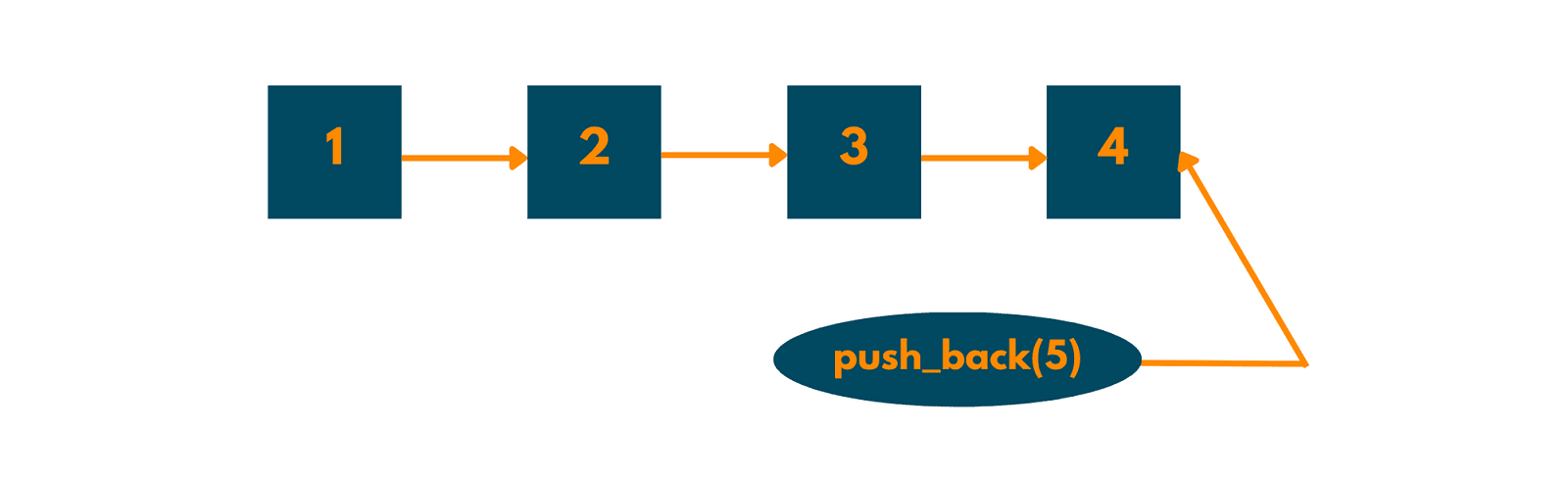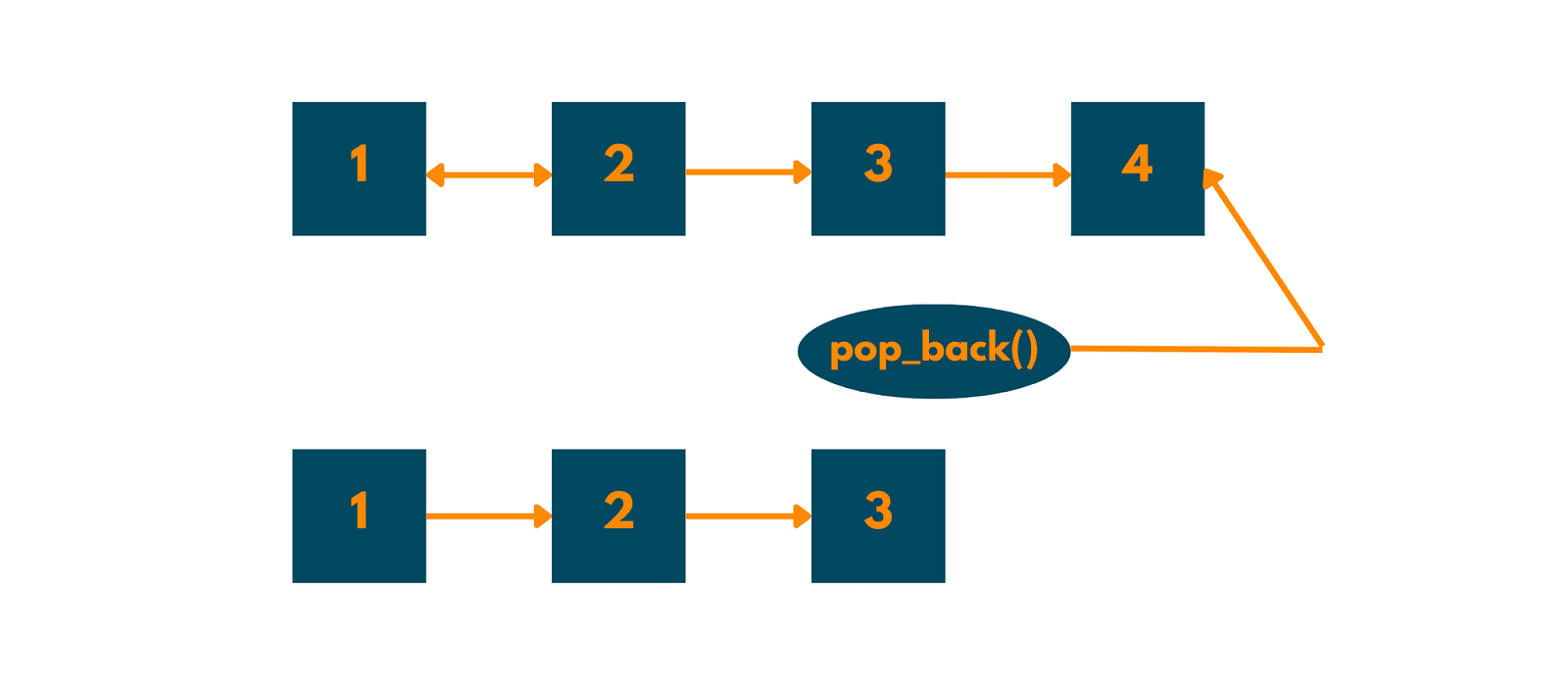# Vector push_back & pop_back Functions in C++ (with Examples)

Vectors in C++ are a part of the standard template library. They are STL Containers that have the unique ability to change their sizes with requirements in order to accommodate insertion and deletion requests inside of the programs. In this article, we will learn about push back operations in vectors with code in C++.

## What is the Vector push_back in C++?

While vectors are similar to dynamic arrays and have the space to adjust their size automatically whenever a particular element is added or removed. Moreover, the storage is easily handled by the container itself.

The vector push_back is a pre-defined function in C++ that helps in inserting elements at the end of the vector. In other words, the push_back function pushes the data or element back into the vector. The container size is increased by 1 and the new value is added at the end of the vector after the current final element. The technique is declared in the header files "vector" and "bits/stdc++.h".

Moreover, every time an element is inserted, the vector’s size increases by one.  Also, the value or data to be inserted or pushed inside the vector is specified by the single parameter that is required by the C++ push_back method and the vector-specific push_back function in C++ has no return value.

The syntax for a push_back function is as follows: vector_name.push_back(element);

Here, the vector name suggests the name of the vector to which an individual wishes to add a particular element or a vector and the element will denote the component’s value.But why do we need them? Here are some of the benefits of push_back in C++. First, you can add elements at the end of the vector. Also, programmers do not have to worry about allocating or deallocating memory because vectors manage memory automatically. And it removes the need for manual memory management and pointer arithmetic, vectors can make your code simpler.

### Example for push_back function:

Here is a small example of the vector push_back operation implemented in C++:

```#include<iostream>

#include<vector>

int main() {

std::vector<int> v;

// Add elements to the vector using push_back()

v.push_back(10);

v.push_back(20);

v.push_back(30);

// Print the vector elements

for (auto i: v) {

std::cout << i << " ";

}

return 0;

}
```

In the above instance, an empty vector v is created. Then, the vector push_back () function is used to extend the vector by three more integers. At last, using the for loop the vector’s value is printed with the result. Therefore, this program’s output would be:

```10 20 30
```

### What is the vector pop_back() in C++?

In C++, the vector pop_back function is used to delete the element from the end of a vector. When this is done, the value is removed from the end of the vector and the container size of the vector is decreased by one.

The syntax for a pop_back function is as follows: vector_name.pop_back();

The vector from which one can remove the final element is identified here by the name vector name.### Example for pop_back() function

Here is a small example of the vector pop_back operation implemented in C++:

```#include<iostream>

#include<vector>

int main() {

std::vector<int> my_vector = {1, 2, 3, 4, 5};

// Print the vector elements

std::cout << "Original vector: ";

for (auto i : my_vector) {

std::cout << i << " ";

}

std::cout << std::endl;

// Remove the last element from the vector using pop_back()

my_vector.pop_back();

// Print the modified vector

std::cout << "Modified vector: ";

for (auto i : my_vector) {

std::cout << i << " ";

}

std::cout << std::endl;

return 0;

}
```

The vector my vector in this example has five integer elements. The vector's elements are then printed using a loop. The last element (5) in the vector is then eliminated from the vector using the pop-back () method. To confirm that the final element was deleted, we finally print the updated vector once more. This program's output would be:

```Original vector: 1 2 3 4 5

Modified vector: 1 2 3 4
```

Keep in mind that the removed element is not returned by the pop-back () function. Before executing pop back, you must access the removed element.

### What can you use instead of Push_back in C++?

Depending on individual needs and program requirements, coders can use the following function instead of the push_back function in C++ to add elements to a vector. Given below are some of them.

You can use Emplace_back() instead of push_back for vectors in C++. This function is similar to push_back(), but the only difference is that it creates an element at the end of the vector instead of copying it. This function may be much more effective because it saves the overhead of duplicating the element when inserting complicated objects into the vectors.

Here's an example of the same:

```std::vector<std::string> my_vector;

my_vector.emplace_back("Hello, World!");
```

There is also the simple insert() function for this purpose. With the help of this method, elements can be added to the vectors at any point and not just at the end. With the help of an iterator, this can be done easily. Here's an illustration:

```std::vector<int> my_vector = {1, 2, 3, 4};

auto it = my_vector.begin() + 2;

my_vector.insert(it, 42);
```

In this instance, the vector has four integer elements. The third element of the vector, with index 2, is subsequently the target of an iterator that we create next. The iterator points to a place, and we use the insert() function to enter the number 42 there. The final vector will look like 1, 2, 42, 3, 4.

At last, we have the resize() function to resize the vectors. It also has the option to initialize the new elements with a certain value. Here's a simple example:

```std::vector<int> my_vector = {1, 2, 3};

my_vector.resize(5, 0);
```

The vector my_vector in this example has three integer elements. We initialize the new elements to 0 and resize the vector to size 5 using the resize() function. 1, 2, 3, 0, 0 will be the final vector. These are only a few instances of C++ methods that can be used in instead of pushback (). Your individual use case and your goals will influence the function you choose.

### What are the exceptions of push_back and pop_back?

Following are some of the exceptions for both these vector operation functions in C++:

• If there is no reallocation of an element already in the vector the container does not change itself in the event of an exception.
• If a reallocation occurs, a strong guarantee is given if the type of an element is copyable or no-throw moveable. Otherwise, the container case will always come to an end in a valid condition that provides a basic guarantee.
• Moreover, undefined behavior can be caused if allocator_traits::construct does not support val as an argument type. In other words, if a vector is null or empty, it shows an undefined behavior.

## The Conclusion

From this article, one can understand that the in-built C++ function is used to push an element into a vector from the back or simply insert data at the end of a vector. Happy Learning :)

### FavTutor - 24x7 Live Coding Help from Expert Tutors!